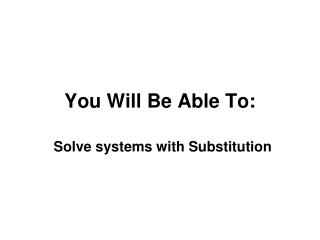Download PresentationYou Will Be Able To:

# You Will Be Able To: - PowerPoint PPT PresentationDownload Presentation## You Will Be Able To:

- - - - - - - - - - - - - - - - - - - - - - - - - - - E N D - - - - - - - - - - - - - - - - - - - - - - - - - - -
##### Presentation Transcript

1. You Will Be Able To: Solve systems with Substitution

2. Solve Systems by Substitution: • Solve for either x or y in one equation • Substitute the expression into the other equation • Solve the equation • Substitute answer back into 1st equation • Solve for other variable • Write answer as ordered pair (x,y)

3. Ex #1: Tell which equation you would use to isolate the variable. Explain your reasoning. 1st one a. x is by itself

4. Ex #1: Tell which equation you would use to isolate the variable. Explain your reasoning. 1st one b. y is by itself

5. Ex #1: Tell which equation you would use to isolate the variable. Explain your reasoning. c. 2nd one Only need to subtract 4x to get “y” by itself

6. Ex #2: Solve the system using substitution y = 3(1) + 2 y = 3 + 2 y = 5 x + 2(3x + 2) = 11 + 4 = 11 x + 6x 7x + 4 = 11 7x = 7 (1, 5) x = 1

7. b. x = -3 + 3 x = 0 y+3 + 2y = -6 3y + 3 = -6 3y = -9 y = -3 (0, -3)

8. c. y = 2(1) + 5 y = 2 + 5 3x + 2x+5 = 10 y = 7 5x + 5 = 10 5x = 5 x = 1 (1, 7)

9. d. x = 1 – 2(1) x = 1 – 2 x = -1 2(1–2y) – 3y = -5 2 – 4y – 3y = -5 -7y + 2 = -5 -7y = -7 y = 1 (-1, 1)

10. Ex #3: Solve the system using substitution x = 2y – 6 x = 2(2) – 6 x = 4 – 6 x = -2 4(2y–6) + 6y = 4 8y – 24 + 6y = 4 14y – 24 = 4 14y = 28 (-2, 2) y = 2

11. b. y = -6x – 1 y = -6(-1) – 1 y = 6 – 1 y = 5 3x + 2(-6x-1) = 7 = 7 – 2 3x – 12x -9x – 2 = 7 -9x = 9 x = -1 (-1, 5)

12. Ex #3: Write a system and solve. During a football game, the parents of the football players sell pretzels and popcorn to raise money for new uniforms. They charge \$2.50 for a bag of popcorn and \$2 for a pretzel. The parents collect \$336 in sales during the game. They sell twice as many bags of popcorn as pretzels. How many bags of popcorn do they sell? x = popcorn = 336 2.50x + 2y y = pretzels x = 2y

13. = 336 2.50x + 2y x = 2y 2(48) x = 96 x = = 336 2.50(2y) + 2y 5y = 336 + 2y 7y = 336 y = 48 96 bags